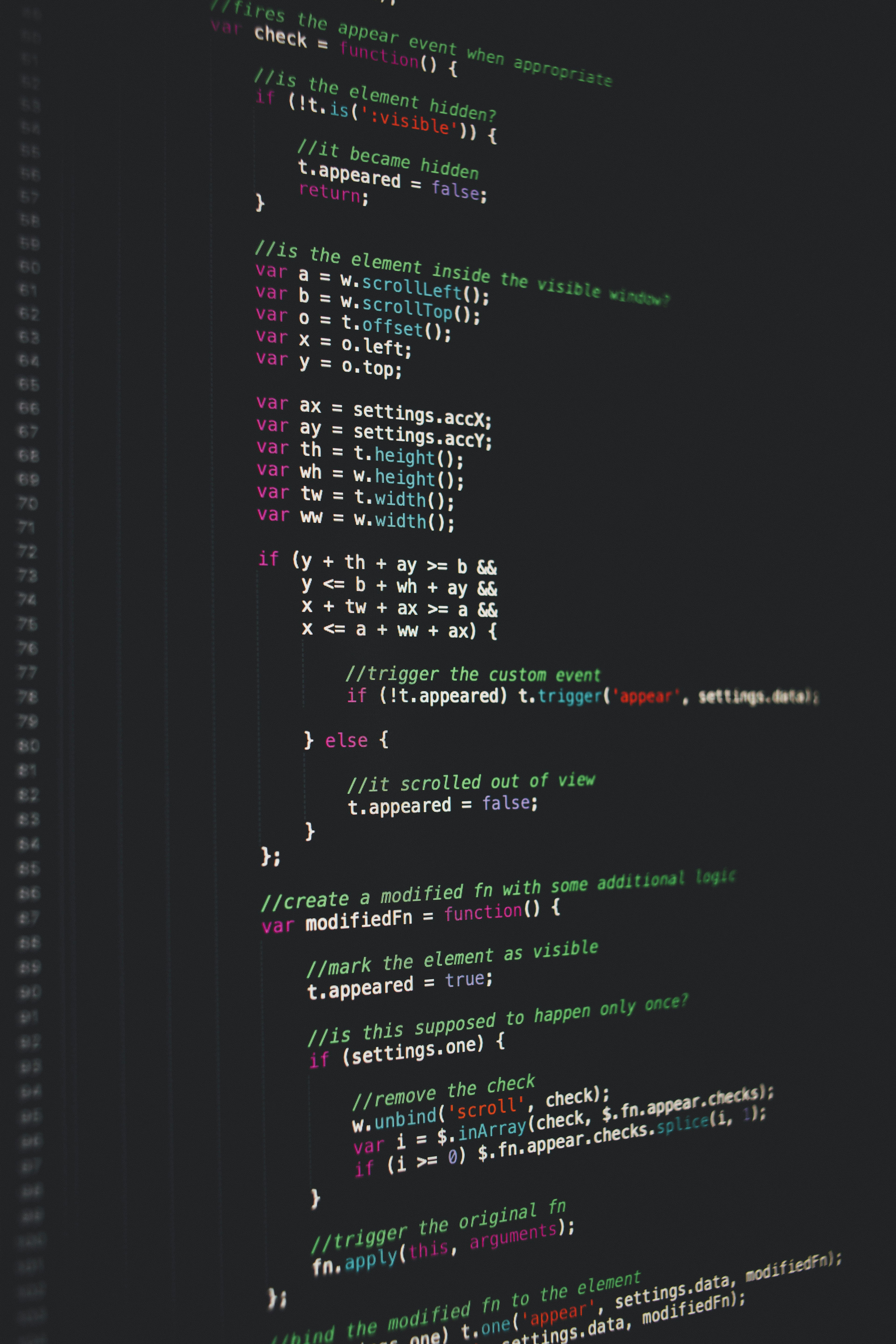Hackerrank Maps-STL Solution

# Hackerrank Maps-STL SolutionMaps are a part of the C++ STL.Maps are associative containers that store elements formed by a combination of a key value and a mapped value, following a specific order.The mainly used member functions of maps are:

Map Template:

std::map <key_type, data_type>


Declaration:

map<string,int>m; //Creates a map m where key_type is of type string and data_type is of type int.


Size:

int length=m.size(); //Gives the size of the map.


Insert:

m.insert(make_pair("hello",9)); //Here the pair is inserted into the map where the key is "hello" and the value associated with it is 9.


Erasing an element:

m.erase(val); //Erases the pair from the map where the key_type is val.


Finding an element:

map<string,int>::iterator itr=m.find(val); //Gives the iterator to the element val if it is found otherwise returns m.end() .
Ex: map<string,int>::iterator itr=m.find("Maps"); //If Maps is not present as the key value then itr==m.end().


Accessing the value stored in the key:

To get the value stored of the key "MAPS" we can do m["MAPS"] or we can get the iterator using the find function and then by itr->second we can access the value.


You are appointed as the assistant to a  teacher in a school and  she is correcting the answer sheets of the students.Each student can have multiple answer sheets.So the teacher has  queries:

:Add the marks  to the student whose name is .

: Erase the marks of the students whose name is .

: Print the marks of the students whose name is .  (If  didn't get any marks print .)

Input Format

The first line of the input contains  where  is the number of queries. The next  lines contain  query each.The first integer,  of each query is the type of the query.If  query is of type , it consists of one string and an integer  and  where  is the name of the student and  is the marks of the student.If query is of type  or ,it consists of a single string  where  is the name of the student.

Constraints

Output Format

For queries of type  print the marks of the given student.

Sample Input

7
1 Jesse 20
1 Jess 12
1 Jess 18
3 Jess
3 Jesse
2 Jess
3 Jess


Sample Output

30
20
0


### Solution in cpp

Approach 1.

#include <cmath>
#include <cstdio>
#include <vector>
#include <iostream>
#include <set>
#include <map>
#include <algorithm>
using namespace std;

int main() {
int n;
cin >> n;
map<string,int>m;
for(int i=0;i<n;i++){
int n1,y;
string z;
cin >> n1 ;
if(n1 == 1){
cin >> z >> y;
if(!m.empty())
m[z] +=y;
else
m.insert(make_pair(z,y));
}
else if(n1==2){
cin >> z;
m.erase(z);
}
else{
cin >> z;
cout << m[z] << endl;
}
}
/* Enter your code here. Read input from STDIN. Print output to STDOUT */
return 0;
}


Approach 2.

#include <cmath>
#include <cstdio>
#include <vector>
#include <iostream>
#include <set>
#include <map>
#include <algorithm>
using namespace std;

int main() {
map <string,int> m;
map <string,int>::iterator iter;
int n, qtype, val;
string key;

cin >> n;
for (int i = 0; i < n; i++) {
cin >> qtype >> key;
if (qtype == 1) {
cin  >> val;
iter = m.find(key);
if (iter == m.end())
m.insert(make_pair(key,val));
else
m[key] += val;
} else if (qtype == 2) {
m.erase(key);
} else if (qtype == 3) {
cout << m[key] << "\n";
}
}
return 0;
}


Approach 3.

#include <cmath>
#include <cstdio>
#include <vector>
#include <iostream>
#include <set>
#include <map>
#include <algorithm>
using namespace std;

int main() {
/* Enter your code here. Read input from STDIN. Print output to STDOUT */
map<string,int>m;
int n;
cin>>n;
while(n-->0){
int cmd;
string name;
cin>>cmd>>name;
if(cmd==1){
std::pair<std::map<string,int>::iterator,bool> ret;
int score;
cin>>score;
ret = m.insert(std::pair<string,int>(name,score));
if(ret.second == false){
ret.first->second = ret.first->second + score;
}
}
if(cmd==2){
m.erase(name);
}
if(cmd==3){
cout<<m[name]<<endl;
}
}
return 0;
}